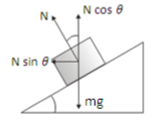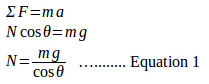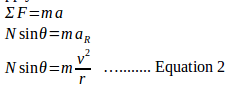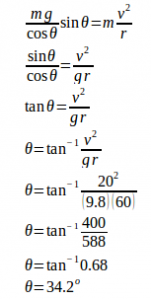# Rounding a banked curve – dynamics of circular motion problems and solutions

1. A car rounding a banked curve. What is an angle for the road which has a curve of radius 60 meters with a design speed of 20 m/ s? Assume there is no friction between car and road.

SolutionN = normal force

N sin θ = horizontal component of the normal force

N cos θ = vertical component of the normal force

w = m g = the weight of the car

The road is designed to be banked to eliminate dependence on friction.

The net horizontal force, the horizontal component of the normal force (N sin θ), required to keep the car moving in a circle around the curve.

We choose x-axis as horizontal and y-axis as vertical, so that centripetal acceleration, aR, is along the horizontal direction. In the horizontal direction, the only force is the horizontal component of the normal force (N sin θ), needed to produce the centripetal acceleration. N sin θ = centripetal force.

Apply Newton’s law of motion in the vertical direction :[irp]

Apply Newton’s law of motion in the horizontal direction :Substituting N in equation 1 into N in equation 2 :[wpdm_package id=’497′]